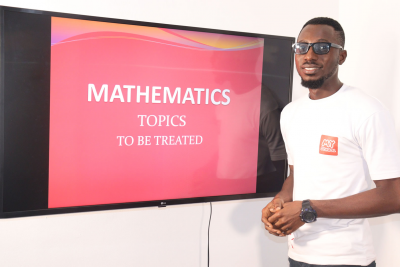### Mathematics video lessons for JAMB, WAEC, NECO and NABTEB candidates + SS1, SS2 and SS3 students

#### Price: ₦1,000

##### Description

Get in-depth, detailed topic by topic video lessons on Mathematics, specially designed for candidates preparing for exams such as JAMB, WAEC, NECO and NABTEB candidates. These video lessons attempt to cover all syllabus in these exams, and are therefore also useful to students in senior secondary schools, SS1, SS2 and SS3. Becoming a full student of this math class also gives you direct access to interact with your tutor on any issue you face in any videos.

PROMO price is currently N1,000 but it will definitely be reviewed upwards after this promo in a few weeks. The best time to buy this subscription is right now, especially if you're preparing for any exam that includes math.

Subscribe for this package right now if you intend to know the principles, and thrash any question problems, in the following maths topics;

• Algebra
• Simple Operations On Algebraic Expression
• Solution Of Linear Equations
• Change Of Subject Of Formula
• Graph Of Linear and Quadratic Functions
• Linear Inequalities
• Algebraic Fractions
• Functions and Relations
• Polynomials
• Progressions
• Binary Operations
• Geometry and Trigonometry
• Angles and Intercepts on Parallel Lines
• Triangles and Polygons
• Loci
• Circles
• Construction
• Coordinate Geometry of Straight Lines
• Trigonometric Ratios
• Angles of Elevation and Depression
• Bearings and Distances
• Number and Numeration
• Number Bases
• Indices
• Logarithms
• Surds
• Fractions, Decimals, Percentages and Approximation
• Sets
• Logical Reasoning
• Modular Arithmetic
• Positive and Negative Integers
• Rational Numbers
• Matrices and Determinants
• Ratios, Proportions and Rates
• Financial Arithmetic
• Variations
• Mensuration
• Lengths and Perimeters
• Areas
• Volumes
• Calculus
• Differentiation
• Application of differentiation
• Integration
• Measures of Dispersion
• Statistics
• Representation of data
• Measures of Location
• Permutation and Combination
• Probability##### Key Features

• Multiple new videos weekly
• Very friendly learning mood to impart knowledge
• Ability to easily rewind the class until you understand any topic

₦1,000
₦3,000
₦1,000
₦3,000